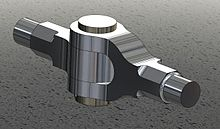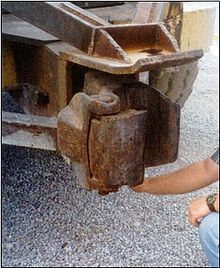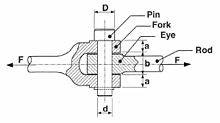# Mechanical joint

Mechanical joint

A mechanical joint is a part of machine which are used to connect the other mechanical part or mechanism. Mechanical joints may be temporary or permanent. Most types are designed to be disassemble when required.

## Types Of Mechanical Joints

1.Knuckle Joints

2.Turnbuckle Joints

3.Pin Joints

4.Cotter Joints

5.Bolted joints

6.Screw Joints

7.Welded Joints

## Knuckle JointsKnuckle joint

A knuckle joint is used to connect the two rods which are under the tensile load, when there is requirement of small amount of flexibility or angular moment is necessary. There is always axial or linear line of action of load.

The knuckle joint assembly consist of following major components :

1.Single eye.

2.Double eye or fork.

3.Knuckle pin.

At one end of the rod the single eye is formed and double eye is formed at the other end of the rod.Both, single and double eye are connected by a pin inserted through eye.The pin has a head at one end and at other end there is a taper pin or split pin. For gripping purpose the ends of the rod are of octagonal forms.Now, when the two eyes are pulled apart, the pin holds them together .The solid rod portion of the joint in this case is much stronger than the portion through which the pin passes.Application of knuckle joint in tractor

The modes of failure are :

1.Shear failure of pin (single shear).

2.Crushing of pin against rod.

3.Tensile failure of flat end bar.

Application :

1.Tie rod joint of roof truss.

4.Tie rod joint of jib crane.

5.The knuckle joint is also used in tractor.

## Design of knuckle joint



The assembly diagram of knuckle joint is as shown in fig.Design of knuckle joint

The dimension of knuckle joints are

Diameter of rod = d

Diameter of knuckle pin = dp

Outside diameter of single eye = doe

Outside diameter of double eye = dod

Thichness of single eye = t

Thickness of fork = t1

Axial tensile force on rod = P

(1) Diameter of rod

Consider the rod is subjected to a direct tensile stress

ς = P /πd2

From above equation ,diameter of rod 'd' is obtained.

(2) Design of pin (dp)

(a) Consider the failure of pin under double shear due to tensile force.

Therefore, direct shear stress induced in knuckle pin is given by Equation

ς= P/ 2A = (P/2) × (Π/4)dp2 = 2P/ Πdp2

(b) Failure of knuckle pin in bending

Assume there is no clearance or slack but in actual, knuckle pin is loose in forks to permit angular moment of one with respect to other, so it is subjected to bending moment in addition to shear, consider uniformly distributed load along the portion of pin.

M = [(-P/2) × (t/4)] + { (P/2) × [ (t2/2)+(t1/3) ] }

= P/2 [(t1/3)+(t/2)-(t1/4) ]

=P/2 [ (t1/3)+(t/4) ]

Section modulus,

Z=(π/ 32)dp3

Maximum bending stress, σb

σb= M/Z = { P/2 [(t1/3)+(t/4)] } / {(π/ 32)dp3}

Here,we check the pin in bending and find the value of dp

(3) Design of single eye :

(a) To find the outside diameter diameter of single eye (doe) The single eye is subjected to a direct tensile stress, due to this single eye under tear.

σt = P/A = P/ (doe-dp)× t

(b) Due to direct tensile strength, the single eye is subjected to double shear.

Resisting shearing area = 2(doe-dp)×(t/2)

The direct shear stress induced is

ς=P/(doe-dp)×t

From this equation the outside diameter of single eye doe is obtained.

(C) Failure of single eye or pin due to tensile load in crushing

Resisting crushing area = dp × t

σc = P/(dp×t)

Form this equation crushing stress checked if fail, increase the thickness of eye (t).

(4) Design of fork (double eye):

(a) The tearing of the double eye at weakest section due to tension

Area resisting tear = (dof – dp) × 2 t1

σt = p/ [(dof – dp) × 2 t1]

From this equation, find the outside diameter of fork (dof).

(b) Failure of double eye (fork) in double shear due to tensile load.

Area resisting shear = 4 × [(dof – dp) ]/2 × t1

= 2 × (dof – dp) t1

The shear stress is given by,

ς = p/[(dof – dp) × 2 t1]

From this equation ,check shear stress if less than design, increase thickness of fork t1.

(c) Failure double eye in crushing (thickness of fork)

Double eye may fail in crushing due to tensile load

The crushing stress is given by,

σc = P/( 2×dp ×t1)

Check crushing stress or find t1

## TURN BUCKLE

The buckle or a coupler is a mechanical joint used to connect two members which are subjected to tensile loading which require slight adjustment of length or tension under loaded conditions. It consist of central hexagonal nut called coupler and tie rod having right hand and left hand threads. A coupler of hexagonal shape is to facilitate the turning of it with a spanner or sometime a hole is provide in the nut so that tommy bar can be inserted for rotating it. As coupler rotate, the tie rod are either pulled together or pushed apart depending upon the direction of the rotation coupler normally the tie rods are made of steel, while coupler is made of steel or C.I.

Application :

1. To tighten the members of the roof truss.

2. Used to connect link in a mechanism to transfer motion

3. Used between the two railway wagon or bogies.

4. To tighten the cable or stay ropes of electric distribution poles.

## Design of Turn Buckle



Consider a turnbuckle , subjected to an axial tensile force P. The various dimensions of the turnbuckle are calculated as follows:

(1) Diameter of tie rod ‘dc2 :

Due to axial tensile load p, the rod is subjected to tensile stress. Also due to twisting moment in the tie rod.

The tie rod is design for direct tensile load of Pd=1.25P

The tensile stress induced in tie rod is given by

σt = 4Pd/ (π×dc×dc)

From above equation the core diameter of the tie rod is obtained. The nominal diameter can be selected from I.S.O metric screw threads table or it can be found by,

do = dc/ 0.84

(2) Length of couple nut (ln) :

Consider the direct shearing of the thread at the root of the coupler nut and the screw.

(a) The direct shear stress induced in screw thread is

ςs = P/(π×dc×ln)

(b) The direct shear stress induced in nut

ςn = P/ (π×do×ln)

Where,

ςs and ςn = shear stress in screw and nut respectively in N/mm2

do = Nominal diameter of the screw

dc = Core diameter of the screw

ln = Length of the coupler nut

(c) Check of crushing of the thread

crushing resistance of the thread = (π/4) × [do2- dc2] ×n×ln× σc

Where,

n = number of threads per length = ln/p

σc = crushing stress induced in the coupler

p = pitch of the thread in mm

Equating the design load with crushing resistance

pd = (π/4) × [do2- dc2] ×n×ln× σc

(3) Outside diameter of the coupler nut (D) :

The coupler nut is subjected to a direct tensile load P and a torque T.

Consider tearing of the coupler nut due to tensile stress induced in it. In order to account for the torque, the tensile load is taken as Pd.

The tensile stress induced in coupler nut is given by

σt = 4P/(π×(D2- do2))

By empirical relation, the outside diameter of the coupler nut D is taken as

D = 1.25 do or 1.5 do

(4) Outside diameter of coupler (D2):

Let,D1= Inside diameter of the coupler

D2 = Outside diameter of the coupler

Consider a coupler is subjected to direct tensile load P and a torque T.So for design the coupler outside diameter ,the design load is consider.

By empirical relation ,

The outside diameter of the coupler D2 is taken as,

D2 = 1.5do or 1.75 do

(5) Length of the coupler nut (Ln)

Ln = 6 do

(6) Thickness of the coupler = t = 0.75 do

(7)Thickness of the coupler nut = t1 = 0.5 do

## COTTER JOINT

cotter joint is used to connect the two member which are subjected to the various stress. The end of the bar has a hole in it and it is called a lug. The hole carries a shaft. This shaft is locked in place by a smaller pin that passes through the side of the lug and partly or completely through the shaft itself. This locking pin is named a cotter, which sometimes is also applied to the whole joint.so this joint is called as a cotter joint.. A cotter joints is a flat wedge link piece of steel of rectangular cross section which is inserted through the rods at high angle to their axes .It is uniform in thickness but tapering in width , generally on one side only. Usually the taper is 1 in 30. when a special arrangement like a set-screw is provided for keeping the cotter from slackening ,its taper may be as large as 1 in 7. the end of the cotter are made narrow to facilitate the hammering for fixing and removing.cotter joins are generally use to fasten rigidly two rod s which is subjected to tensile or compressive stress along their axes. this joint is used to connect two circular rods.This joint in not suitable where the member are subjected under rotation.Thus they differ from key joints which are used to fasten shaft and hubs subjected to tensional stress.

APPLICATIONS OF COTTER :

1.Connection of the piston rod with the cross heads 2.Joining of tail rod with piston rod of a wet air pump 3.Foundation bolt 4.Connecting two halves of fly wheel (cotter and dowel arrangement) COMPARISON BETWEEN KEY AND COTTER

1.Key is usually driven parallel to the axis of the shaft which is subjected to torsional or twisting stress. Whereas cotter is normally driven at right angles to the axis of the connected part which is subjected to tensile or compressive stress along its axis. 2.A key resists shear over a longitudinal section whereas a cotter resist shear over two transverse section.

DIFFERENT TYPES OF COTTER JOINTS

1.Socket and spigot cotter joint 2.Sleeve and cotter joint 3.Gib and cotter joint

Wikimedia Foundation. 2010.

### Look at other dictionaries:

• Joint (geology) — Joint sets on a bedding plane in flagstones, Caithness, Scotland …   Wikipedia

• joint — joint, articulation, suture denote a place where two things are united or the mechanism by which they are united. Joint is the most inclusive of these terms and is freely usable in reference both to anatomical and mechanical structures. In… …   New Dictionary of Synonyms

• Mechanical Animals Tour — by Marilyn Manson Associated album Mechanical Animals Start date Octob …   Wikipedia

• Mechanical Cabaret — are a British synthpop project founded by the musician Roi Robertson, based in North London, and signed to the German record label Major Records Medien GmbHand published by Warner/Chappell Entertainment GmbH. Contents 1 Early Years: 1999 2004… …   Wikipedia

• joint disease — Introduction       any of the diseases or injuries that affect human joints (joint). arthritis is no doubt the best known joint disease, but there are also many others. Diseases of the joints may be variously short lived or exceedingly chronic,… …   Universalium

• joint — /joynt/, n. 1. the place at which two things, or separate parts of one thing, are joined or united, either rigidly or in such a way as to permit motion; juncture. 2. a connection between pieces of wood, metal, or the like, often reinforced with… …   Universalium

• Mechanical powder press — Contents 1 Function Principles of mechanical presses in the field of Technical Ceramics 2 Application Advantages in the field of Technical Ceramics 3 Context between ceramic compaction …   Wikipedia

• Joint manipulation — For extended detail of manipulation of spinal joints, see Spinal manipulation. Joint manipulation is a type of passive movement of a skeletal joint. It is usually aimed at one or more target synovial joints with the aim of achieving a therapeutic …   Wikipedia